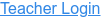PhET Interactive Simulations

### Topics

• Kinematics
• Air Resistance
• Parabolic Curve
• Vectors
• Drag Force
• Projectile Motion

### Description

Blast a car out of a cannon, and challenge yourself to hit a target! Learn about projectile motion by firing various objects. Set parameters such as angle, initial speed, and mass. Explore vector representations, and add air resistance to investigate the factors that influence drag.

### Sample Learning Goals

• Determine how each parameter (initial height, initial angle, initial speed, mass, diameter, and altitude) affects the trajectory of an object, with and without air resistance.
• Predict how varying the initial conditions will affect a projectile’s path, and provide an explanation for the prediction.
• Estimate where an object will land, given its initial conditions.
• Determine that the x and y motion of a projectile are independent.
• Investigate the variables that affect the drag force.
• Describe the the effect that the drag force has on the velocity and acceleration.
• Discuss projectile motion using common vocabulary (such as: launch angle, initial speed, initial height, range, time).
Version 1.0.3